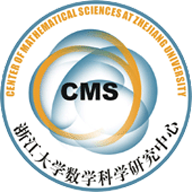(12011)GEOMETRIC, TOPOLOGICAL AND DIFFERENTIABLE　RIGIDITY OF SUBMANIFOLDS IN SPACE FORMS

 AbstractLet M be an n-dimensional submanifold in the simply connected space form　Fn+p(c) with c + H2 > 0, where H is the mean curvature of M. We verify that　if Mn(n ≥ 3) is an oriented compact submanifold with parallel mean curvature and　its Ricci curvature satisfies RicM ≥ (n−2)(c+H2), then M is either a totally umbilic　sphere, a Clifford　hypersurface in an (n+1)-sphere with n = even, or CP2( 4　3 (c+H2))in S7(p 1　c+H2 ). In particular, if RicM > (n − 2)(c + H2), then M is a totally umbilic　sphere. We then prove that if Mn(n ≥ 4) is a compact submanifold in Fn+p(c) with　c ≥ 0, and if RicM > (n−2)(c+H2), then M is homeomorphic to a sphere. It should　be emphasized that our pinching conditions above are sharp. Finally, we obtain a　differentiable sphere theorem for submanifolds with positive Ricci curvature.

• Xu-Gu.pdf